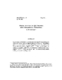## Error Analysis of QR Updating with Exponential Windowing##### Files
CS-TR-2685.ps(121.33 KB)
To appear in Mathematics of Computation Exponential windowing is a widely used technique for suppressing the effects of old data as new data is added to a matrix. Specifically, given an $n\times p$ matrix $X_n$ and a forgetting factor'' $\beta\in(0,1)$, one works with the matrix $\dia(\beta^{n-1},\beta^{n-2},\ldots,1)X_n$. In this paper we examine an updating algorithm for computing the QR factorization of $\dia(\beta^{n-1},\beta^{n-2},\ldots,1)X_n$ and show that it is unconditionally stable in the presence of rounding errors. (Also cross-referenced as UMIACS-TR-91-79)